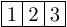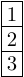Queries for Standard tableaux: search statistic / browse statistics / browse maps from / browse maps to

# 1. Definition & Example

• A standard (Young) tableau of a partition $\lambda \vdash n$ is a bijection between $\{1,2,\dots, n\}$ and the cells (also known as boxes) of the Young diagram of $\lambda$, such that rows and columns are increasing.

• The set of standard tableaux of size $n$ is denoted by $\mathcal{SYT}_n$ where the size is given by the size of the underlying partition $\lambda \vdash n$.

 the 4 Standard tableaux of size 3[[1,2,3]] [[1,3],] [[1,2],] [,,]
• Standard tableaux are graphically represented by filling the cells of the Young diagram in English notation.

# 2. Properties

• The number of standard tableaux of a partition $\lambda$ is given by the well known hook-length formula $$\big|\mathcal{SYT}_\lambda\big| = \frac{n!}{\prod hook(B)},$$ where the product ranges over all boxes $B$ in the Young diagram of $\lambda$, and where $hook(B)$ is the hook length of $B$ in $\lambda$. The hook length of $B$ is the number of boxes in the same row to the right of $B$, plus the number of boxes in the same column below $B$ plus the box itself.

• The number of standard tableaux of size $n$ cells is counted by the involution numbers, with the first few terms (beginning with $n=0$) being 1, 1, 2, 4, 10, 26.

# 3. Remarks

• The number of standard tableaux of shape $(n,n)$ is counted by the $n^{th}$ Catalan number, $\operatorname{C}_n = \frac{1}{n+1} \binom{2n}{n}$.

• There is a bijection between permutations $\pi \in \mathbf{S}_n$ and pairs $(P,Q)$ of Standard Tableaux of shape $\lambda \vdash n$, known as the Robinson–Schensted correspondence. Furthermore, the length of the longest increasing subsequence of $\pi$ is equal to the length of the first row of $P$ (or $Q$), and the length of the longest decreasing subsequence of $\pi$ is equal to the height of the first column of $P$. [Sch61]

• There is a bijection between Standard Young Tableaux of shape $(3×n)$ and irreducible webs for ${sl_3}$ whose boundary vertices are all sources [Tym12]

[Sch61]   C. Schensted, Longest increasing and decreasing subsequences, Canad. J. Math. 13(1961), 179-191.

[Tym12]   Julianna Tymoczko, A simple bijection between standard 3×n tableaux and irreducible webs for sl3, J Algebr Comb (2012) 35:611–632.

# 6. Technical information for database usage

• A standard tableau is uniquely represented as a list of lists giving the fillings of the cells row by row.
• Standard tableaux are graded by the size.
• The database contains all standard tableaux of size at most 8.